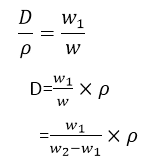Mechanics

# Archimedes principle and buoyant force

Archimedes’ principle states that when an object is totally or partially immersed in a liquid, an upthrust acts on it equal to the weight of the liquid it displaces. Boats and ships floating on the surface of the water are examples of the Archimedes principle. Ships work on the principle of flotation.

An air-filled balloon immediately shoots up to the surface when released underwater. The same would happen if a piece of wood is released underwater. We might have noticed that a mug filled with water feels light underwater but feels heavy as soon as we take it out of the water.

More than two thousand years ago, the Greek scientist, Archimedes noticed that there is an upward force that acts on an object kept inside a liquid. As a result, an apparent loss of weight is observed in the object is called the upthrust of the liquid.

## Archimedes principle formula

Consider a solid cylinder of cross-sectional area A and height h immersed in a liquid. Let h1 and h2 be the depths of the top and bottom faces of the cylinder respectively from the surface of the liquid.
Then :

h2 – h2 = h
If P1 and P2 are the liquid pressure at depths h1 and h2 respectively and ρ is the density, then according to equation (2):

P1 = ρ g h 1

P2 = ρ g h2

Then the force F1 is exerted at the cylinder top by the liquid due to pressure P1 and the force F2 is exerted at the bottom of the cylinder by the liquid due to P2.

F1 = PA = ρ g hA

and   F2  = P2 A = ρ g h2 A

Fand F2 are acting on opposite faces of the cylinder. Therefore, the net force F will be F2 – F in the direction of F2. This net force F on the cylinder is called the upthrust of the liquid.

F– F=ρ g h2 A – ρ g hA

=ρ gA(h– h1)

Upthrust of liquid =ρ gAh

or                                        =ρ gV

Here Ah is the volume V of the cylinder and is equal to the volume of the liquid displaced by the cylinder. Therefore, ρ g V is the weight of the liquid displaced. In the equation that an upthrust acts on the body immersed in a liquid and is equal to the weight of the liquid displaced, is Archimedes law.

## Density Of an Object by Archimedes law

Archimedes’s law is also helpful to determine the density of an object. The ratio in the weights of a body with an equal volume of liquid is the same as in their densities.

Let the Density of the object = D
The density of the liquid  = ρ
Weight of the object   = w1
Weight of equal volume of liquid = w = w1 – w2

Here w is the weight of the solids in a liquid. According to Archimedes’ law, w2 is less than its actual weight w1 by an amount of w.Thus finding the weight of the solid in air w, and its weight in water w2, we can calculate the density of the solid by the above equation.

watch also video:

## Principle Of Floatation

A floating object displaces a fluid having a weight equal to the weight of the object.
An object sinks if its weight is greater than the upthrust acting on it. An object floats if its weight is equal or less than the upthrust. When an object floats in a fluid, the upthrust acting on it is equal to the weight of the object. In the case of a floating object, the object may be partially immersed. The upthrust is always equal to the weight of the fluid displaced by the object. This is the principle of floatation.